# Stock return analysis using histograms & 4 skewness of histograms

A histogram is a widely used way to summarize a large data set. Creating a histogram using Data Analysis ToolPak is very easy. My this blog is aimed to show you how you can use a histogram chart to summarize the returns of a stock. Then I will show you how you can compare the returns of two or more stocks using their histogram charts.

## Creating a histogram using stock returns data

In the image below, you are seeing total 4 stocks with their monthly returns. The stocks are Microsoft, General Electric, Intel Corporation, General Motors, and Cisco. The leftmost column is showing the last working day of the month, and the date starts from March 30, 1990.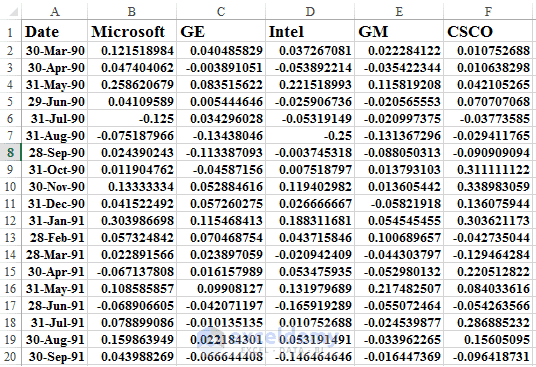Returns of 5 stocks.

There are total 130 data points in a column, from March 30, 1990, to December 29, 2000. A data set with 130 data points is not that large, but it is ok for our analysis. If you want to add more data points for your analysis, you can visit any website that stores daily stock price.

Before calculating the return on a stock, let’s revise again the general rules of building a histogram from a large data set.

### Steps to Create a Histogram

• Find out the minimum value of the data set (use Excel’s MIN() function)
• Find out the maximum value of the data set (use Excel’s MAX() function)
• Find out the difference between these two values: Difference = Maximum – Minimum
• A standard histogram should have a number of ranges between 8 to 15 (my advice is: take total 10 ranges)
• So divide the Difference value by 10 or the number of ranges you have chosen.

### Now we shall make a histogram using our Cisco data.

1. Open the Data Analysis dialog box (Data tab → Analysis group of commands → Data Analysis)
2. From the Data Analysis dialog box, choose Histogram option. Histogram dialog box will appear.
3. In the Input range, insert cell range \$F\$1:\$F\$131, in the bin range insert cell range \$I\$7:\$I\$14. Select checkbox Labels. As our selected input and bin ranges have labels (CSCO & Bins). As Output range, I select cell reference: \$H\$16. And finally, I select the Chart Output checkbox as I want to see the Histogram also on the chart.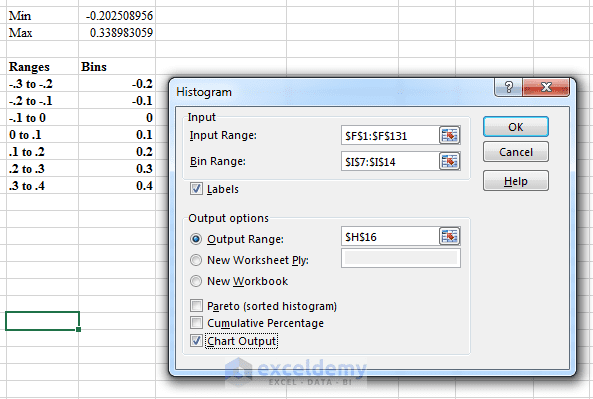Steps of creating a histogram using Data Analysis ToolPak.

4. When done, just click OK. You will get a Histogram table and chart like shown in the image below.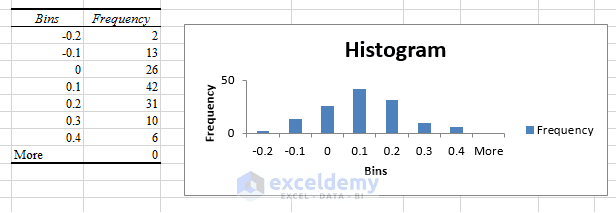Cisco stock histogram with chart

So, this is our frequency distribution table with the chart output. From the frequency table and from the chart, it is clear that Cisco returns are most likely between 0 and 10 percent per month and the height of the bars drops off as the graph moves away from the tallest bar.

## Stock returns comparison using histograms

Now, you will see how to use histograms to compare two stocks.

Analysts are often asked to compare different datasets. For example, you might be asked how the monthly returns on GM and Cisco stock differ. To answer a question such as this, you can construct a histogram for GM by using the same bin ranges as for Cisco, then place one histogram above the other.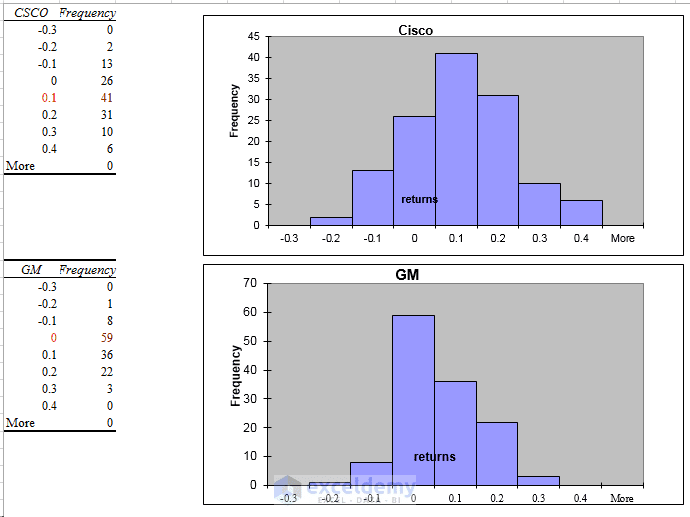Stock Returns Comparison

In the screen, you are seeing, I have constructed the histograms of Cisco and GM using the same bin ranges -.3 to -.2, -.2 to -.1 and so on.

By comparing these two histograms, you can draw two important conclusions:

1. Typically, Cisco performed better than GM. Because the highest bar for Cisco is one bar to the right of the highest bar for GM. Also, the Cisco bars extend farther to the right than the GM bars. GM returns are up to 30% when the Cisco returns are up to 40%.
2. Cisco had more variability or spread about the mean, than GM. Note that GM’s peak bar contains 59 months, whereas Cisco’s peak bar contains only 41 months. This shows that for Cisco, more of the returns are outside the bin that represents the most likely Cisco return. Cisco returns are more spread out than GM returns.

So as a stock analyst, you should go for Cisco, not General Motors.

## What are some common shapes of histograms?

For most data sets, a histogram created from the data will be classified as one of the following:

1. Symmetric
2. Skewed right (positively skewed)
3. Skewed left (negatively skewed)
4. Multiple peaks

### Symmetric Distribution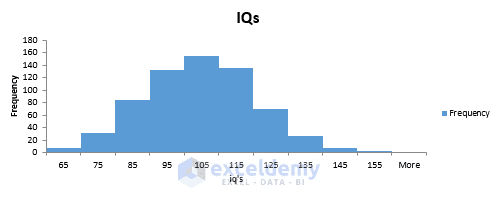Symmetric distributions

• A histogram is symmetric if it has a single peak and looks approximately the same to the left of the peak as to the right of the peak.
• Test scores (such as IQ tests) are often symmetric.
• Notice that the height of the bars one bar away from the peak bar is approximately the same, the height of the bars two bars away from the peak bar are approximately the same, and so on.

### Skewed right – Positively Skewed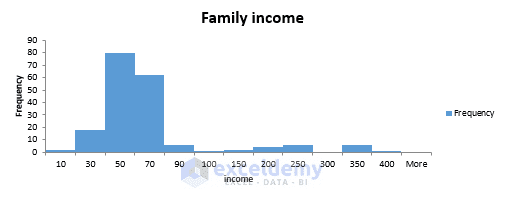Skewed right or positively skewed

• A histogram is skewed right (positively skewed) if it has a single peak and the values of the data set extend much farther to the right of the peak than to the left of the peak.
• Many economic data sets (such as family or individual income) exhibit a positive skew.
• This figure shows an example of a positively skewed histogram created from a sample of family incomes.

### Skewed left – Negatively Skewed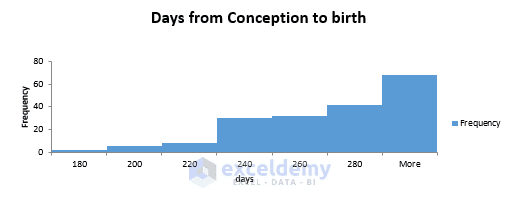Skewed left or negatively skewed

• A histogram is skewed left (negatively skewed) if it has a single peak and the values of the data set extend much farther to the left of the peak than to the right of the peak.
• Days from conception to birth are negatively skewed.
• In the figure, the height of each bar represents the number of women whose time from conception to birth fell in the given bin range.

### Multiple peaks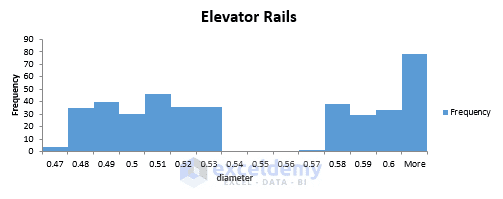Multiple Peaks

• When a histogram exhibits multiple peaks, it usually means that data from two or more populations are being graphed together.
• For example, suppose the diameter of elevator rails produced by two machines yields the histogram shown in the Figure.

Let’s talk more about Twin Peaks or Multiple Peaks histograms.

In this histogram, the data clusters into two groups. In all likelihood, the left group of elevator rails is produced by one machine and the right group of elevator rails are produced by other machines.

If your required diameter for an elevator rail is .55 inches, you can conclude that one machine is producing elevator rails that are too narrow, whereas the other machine is producing elevator rails that are too wide.

And both machines are failed to produce your required .55 inches diameter of elevator rails.

This example shows why histograms are a powerful tool in quality control.

Make Frequency Distribution Table In Excel In 7 Ways

How to Use Wildcards in Excel?

Use Scatter Chart in Excel to Find Relationships between Two Data Series

How to Make a Histogram in Excel Using Data Analysis ToolPak

The Main Differences between a Bar Graph and a Histogram

Stock Return.xlsx

Skewness Examples.xlsx

Multiple Peaks.xlsx

This is a sample lecture of my course: Data Analysis in Excel with Statistics: Get Meanings of Data where you will learn Data Analysis with 52 case studies, problems, and their solutions!

#### Kawser

Hello! Welcome to my Excel blog! It took me some time to be a fan of Excel. But now I am a die-hard fan of MS Excel. I learn new ways of doing things with Excel and share here. Not only how to guide on Excel, but you will get also topics on Finance, Statistics, Data Analysis, and BI. Stay tuned!

1 Comment